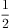### Create an Account

Home / Questions / Consider the following equilibrium

# Consider the following equilibrium

Consider the following equilibrium:

2 H2(g) + S2(g2 H2S(gKc = 1.08 × 107at 700 °C

a) Calculate Kp(b) Does the equilibrium mixture contain mostly H2 and S2 or mostly H2S? (c) Calculate the value of Kc if you rewrote the equation H2(g) +S2(gH2S(g).

Aug 22 2021 View more View LessSubscribe To Get Solution